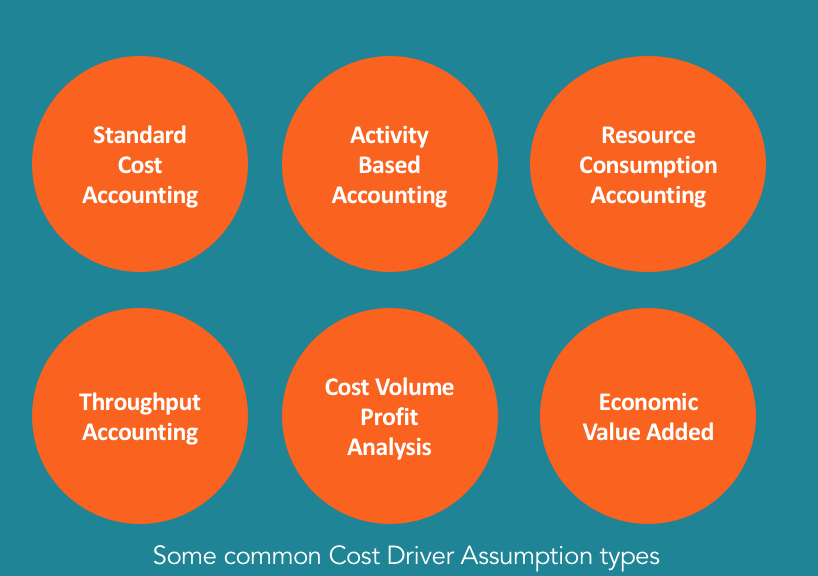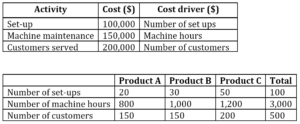# Cost Driver

The direct cause of a cost

## What is a Cost Driver?

A cost driver is the direct cause of a cost, and its effect is on the total cost incurred. For example, if you are to determine the amount of electricity consumed in a particular period, the number of units consumed determines the total bill for electricity. In such a scenario, the number of units of electricity consumed is a cost driver.### Application of a Cost Driver in Computing a Product’s Cost

In a business venture, the major determinant of whether there will be continuity or discontinuity is cost. If the cost of production exceeds the revenue derived from a sale, there is a great probability of the business closing down. If the costs are less than revenue, there is profit and a probability of expansion. If the costs equal revenue, then the business is at a point of indifference and it can be closed or continued depending on other variables apart from cost or on how costs can possibly be adjusted.

In order to make rational business decisions, you require viable costing methods to get the correct cost or a figure which is close enough to the actual cost for you to perform reliable cost/revenue analysis. Failure to do so can lead to the closing of a business venture, due to poor cost computation, that may actually be profitable, or at least potentially profitable.

Total production costs are used to set the selling prices for particular products. Thus, if the costs are inaccurate, the profit forecasts will not be accurate, and the whole accounting system of the particular organization will be subject to errors.

Our main focus here will be Activity-Based Costing (ABC).

### Activity-Based Costing (ABC)

An activity’s costs can be allocated to a particular production lot, and this makes activity-based costing an accurate way of allocating both direct and indirect costs. It is a method of computing costs associated with each product or line of production in a company based on the amount of resources consumed by each activity.

As a result, cost drivers are most relevant in the ABC costing system.  The cost of each activity is apportioned to specific products or lines of production, based on resources consumed by cost drivers. A cost driver is a factor that creates or drives the cost of the activity. It is the root cause of why a particular cost occurred.

Activities consume resources while customers, products, and channels of production consume activities. Understanding this is fundamental to the cost allocation concept using cost drivers. The profitability of each customer can also be easily evaluated using cost drivers, and in cases of resource constraints, the less profitable order can be eliminated. Resources should be allocated to the most profitable activities or in proportion to profitability.

For example, in most operations machines are used and, thus, the machine hours used determines the total cost of operating the machine depending on how much money is charged per hour. If a person operates a machine for 10 hours at a cost of \$10 per hour, then the total cost that will be charged to the output of that particular time is \$100. The more labor hours used, the higher the cost.

If the particular machine we are referring to requires maintenance costing \$1,000 after operating 2,000 hours, then the maintenance cost per every hour of machine operation is 50 cents (\$1.000/2.000 hrs.). Thus, machine hours can be classified as a cost driver.

Another factor that determines total cost is the cost per hour. If the cost per hour is high, then the cost associated with the output will also rise. Many variables determine the cost of production. The indirect costs associated with a line of production, such as quality control costs, are apportioned based on a ratio or a weight based on the products that were subjected to quality control.

The main challenge of ABC costing is that it allocates fixed costs as if they were variable. Because of this fact, it may give an inaccurate figure of the total cost, and the inaccuracy depends on the period of time required to recoup back the initial fixed cost. If the cost is high, there are likely to be lower profits in the first years of operation, and more profit as more costs are absorbed.

Generally, any untraceable cost should be subtracted from the contribution or the operating profit but not allocated to individual products without any logical base.

### Types of Drivers in Cost Accounting

In a traditional system of accounting, the indirect costs or manufacturing overheads are allocated to the production cost based on a predetermined rate. In some accounting systems, cost drivers are almost irrelevant in determining the contribution.

• Number of set-ups
• Number of machine hours
• Number of processed orders
• Number of orders completed
• Number of labor hours
• Number of orders packed and delivered

### Significance of Cost Drivers in Cost Accounting

Whatever determines the total cost of a particular activity should be analyzed in depth to ensure that a proper allocation base is used. Cost drivers follow a cause-effect relationship, and if the relationship cannot be established, then a more relevant driver should be looked for.

### Example of a Cost Allocation Based on Cost Drivers

We are going to look at the following example in order to get a clear picture of how cost drivers are used to derive each product’s or line of production’s total costs.

The following information is for the three lines of production of ABZ Company, which uses Activity-Based Costing:The company plans to produce 300 units of product A, 400 units of product B, and 500 units of product C. Compute the cost per unit of each product.

#### Cost per set-up

Based on the number of set-ups as the basis of allocating set-up cost to products, the cost per set-up will be:

• Total set-up cost = \$100,000
• Total number of set-ups = 100
• Cost per set-up = 100,000/100 = \$1,000
• Set-up cost associated with product A = 1,000 x 20 = \$20,000
• Set-up cost associated with product B = 1,000 x 30 = \$30,000
• Set-up cost associated with product C = 1,000 x 50 = \$50,000

#### Cost per machine hour

• Total cost associated with machine maintenance = \$150,000
• Total number of machine hours = (800+1,000+1,200) = 3,000 hours
• Cost of each hour of machine maintenance = 150,000/3,000 = \$50
• Machine maintenance cost associated with product A = 800 x \$50 = \$40,000
• Machine maintenance cost associated with product B = 1,000 x \$50 = \$50,000
• Machine maintenance cost associated with product C = 1,200 x \$50 = \$60,000

#### Cost associated with each customer served

• Total cost associated with number of customers served = \$200,000
• Total number of customers served = 500
• Cost per each customer served = \$200,000/500= \$400
• Customer service cost associated with product A = 150 x \$400 = \$60,000
• Customer service cost associated with product B = 150 x \$400 = \$60,000
• Customer service cost associated with product C = 200 x \$400= \$80,000

Based on the above cost drivers, the company’s cost can be allocated to the products as follows:

#### Product A

Set-up + Machine Maintenance + Customer Service =

(\$20,000 + \$40,000+ \$60,000) = \$120,000

#### Product B

Set-up + Machine Maintenance + Customer Service =

(\$30,000+ \$50,000+ \$60,000) = \$140,000

#### Product C

Set-up + Machine Maintenance + Customer Service =

(\$50,000 + \$60,000 + \$80,000) = \$190,000

Cost associated with each unit produced

• Cost per unit of product A = Total cost/Number of units = \$120,000/300= \$400
• Cost per unit of product B = \$140,000/400= \$350
• Cost per unit of product C = \$190,000/ 500= \$380

### Key Takeaways

1. A cost driver is the most appropriate way of calculating or determining a specific cost.
2. Variable cost drivers can come in the form of hourly costs, costs per unit, or batch costs, among others.
3. Cost drivers can be fixed costs, such as in the case of set-up costs.

CFI is the official provider of the global Financial Modeling & Valuation Analyst (FMVA)™ certification program, designed to help anyone become a world-class financial analyst. To keep advancing your career, the additional CFI resources below will be useful:

• Target Costing
• Fixed and Variable Costs
• Financial Accounting Theory
• Guide to Financial Modeling